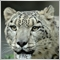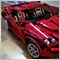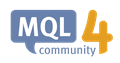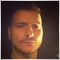# Indicator keeps updating already counted data.893

Hello everyone,

Here is the code below. Where I have I allowed this to occur.

```#property copyright "Copyright 2018, MetaQuotes Software Corp."
#property link      "https://www.mql5.com"
#property version   "1.00"
#property strict
#property indicator_separate_window
#property indicator_minimum 0
#property indicator_maximum 100
#property indicator_buffers 9

extern ENUM_TIMEFRAMES TIME_FRAME=PERIOD_H1;
long chartid,Chart_ID;
double Buffer[],Buffer1[],Buffer2[],Buffer3[],Buffer4[],Buffer5[],Buffer6[],Buffer7[],Buffer8[];
int i,k=0,counted_bars=0;
double A,B,C,D,E,F,G,H,x;
//+------------------------------------------------------------------+
//| Custom indicator initialization function                         |
//+------------------------------------------------------------------+
int init()
{
bool T=ChartSetSymbolPeriod(Chart_ID,Symbol(),TIME_FRAME); ChartSetInteger(Chart_ID,CHART_SHOW_PERIOD_SEP,false);
ChartSetInteger(Chart_ID,CHART_SHIFT,4);                   ChartSetInteger(Chart_ID,CHART_COLOR_CANDLE_BEAR,clrCoral);
ChartSetInteger(Chart_ID,CHART_SHOW_GRID,false);           ChartSetInteger(Chart_ID,CHART_COLOR_CANDLE_BULL,clrBlue);
ChartSetInteger(Chart_ID,CHART_SHOW_OHLC,false);           ChartSetInteger(Chart_ID,CHART_COLOR_CHART_DOWN,clrCoral);
ChartSetInteger(Chart_ID,CHART_SCALE,3);                   ChartSetInteger(Chart_ID,CHART_COLOR_CHART_UP,clrBlue);
ChartSetInteger(Chart_ID,CHART_COLOR_ASK,clrGreen);        ChartSetInteger(Chart_ID,CHART_COLOR_BACKGROUND,clrWhite);
ChartSetInteger(Chart_ID,CHART_COLOR_BID,clrBlack);        ChartSetInteger(Chart_ID,CHART_AUTOSCROLL,true);
ChartSetInteger(Chart_ID,CHART_SHOW_ASK_LINE,true);        ChartSetInteger(Chart_ID,CHART_COLOR_BID,clrBlack);
ChartSetInteger(Chart_ID,CHART_MODE,1);                    ChartSetInteger(Chart_ID,CHART_COLOR_FOREGROUND,clrBlack);
SetIndexStyle(0,DRAW_LINE,1,2,clrBlue);   SetIndexBuffer(0,Buffer);
SetIndexStyle(1,DRAW_LINE,1,2,clrGreen);  SetIndexBuffer(1,Buffer1);

return(INIT_SUCCEEDED);
}
//+------------------------------------------------------------------+
int start()
{
for(i=0; i<400; i++)
{
A[i]  =iStochastic(Symbol(),PERIOD_M1,5,2,2,MODE_SMA,1,MODE_MAIN,i);
B[i]  =iStochastic(Symbol(),PERIOD_M5,5,3,3,MODE_SMA,1,MODE_MAIN,i);
C[i]  =iStochastic(Symbol(),PERIOD_M15,5,5,5,MODE_SMA,1,MODE_MAIN,i);
D[i]  =iStochastic(Symbol(),PERIOD_M30,5,7,7,MODE_SMA,1,MODE_MAIN,i);
A[i]  =iStochastic(Symbol(),PERIOD_M1,21,7,7,MODE_SMA,1,MODE_MAIN,i);
F[i]  =iStochastic(Symbol(),PERIOD_H4,5,9,9,MODE_SMA,1,MODE_MAIN,i);
G[i]  =iStochastic(Symbol(),PERIOD_W1,14,3,3,MODE_SMA,1,MODE_MAIN,i);
H[i]  =iStochastic(Symbol(),PERIOD_MN1,10,3,3,MODE_SMA,1,MODE_MAIN,i);
x=(A[i]+B[i]+C[i]+D[i]+E[i]+F[i]+G[i]+H[i])/8;
Buffer[i]=MathSqrt(A[i])+MathSqrt(B[i])+MathSqrt(C[i])+MathSqrt(D[i])+MathSqrt(E[i])+MathSqrt(F[i])+MathSqrt(G[i])+MathSqrt(H[i]/8);
Buffer1[i]=x;
}
return(0);
}
//+------------------------------------------------------------------+
```

I have found that both Buffers are changing past values to what I  think are relative to the current values. How do I correct the code to only update the current incoming data only?393

GrumpyDuckMan:

Hello everyone,

Here is the code below. Where I have I allowed this to occur.

I have found that both Buffers are changing past values to what I  think are relative to the current values. How do I correct the code to only update the current incoming data only?

Check this out : https://docs.mql4.com/customind/indicatorcountedIndicatorCounted - Custom Indicators - MQL4 Reference
• docs.mql4.com
The most calculated bars do not need any recalculation. In most cases, same count of index values do not need for recalculation. The function is used to optimize calculating.29724

Try these  , you will have to also work on the data you are referencing by the way . an Open of H1 is not the same Open as MN1 .

```//+------------------------------------------------------------------+
//|                                             MultiStochastics.mq4 |
//|                                @Copyright 2019 Lorentzos Roussos |
//|                              https://www.mql5.com/en/users/lorio |
//+------------------------------------------------------------------+
#property copyright "@Copyright 2019 Lorentzos Roussos"
#property link      "https://www.mql5.com/en/users/lorio"
#property version   "1.00"
#property strict
#property indicator_separate_window
#property indicator_buffers 2
#property indicator_plots   2
//Training #651
//--- plot SquareRoot
#property indicator_label1  "SQRT"
#property indicator_type1   DRAW_LINE
#property indicator_color1  clrDarkOrange
#property indicator_style1  STYLE_SOLID
#property indicator_width1  1
//--- plot Average
#property indicator_label2  "AVG"
#property indicator_type2   DRAW_LINE
#property indicator_color2  clrMediumSeaGreen
#property indicator_style2  STYLE_SOLID
#property indicator_width2  1

input ENUM_TIMEFRAMES TIME_FRAME=PERIOD_H1;//TimeFrame:
input int MaxCalculation=400;//MaxBars
//--- indicator buffers
double SQRT[];
double AVG[];

datetime barstamp=0;
bool FirstCalculation=false;
//+------------------------------------------------------------------+
//| Custom indicator initialization function                         |
//+------------------------------------------------------------------+
int OnInit()
{
long Cid=ChartID();
bool t=ChartSetSymbolPeriod(Cid,Symbol(),TIME_FRAME);
if(t==false) return(false);
//--- indicator buffers mapping
SetIndexBuffer(0,SQRT);
SetIndexBuffer(1,AVG);
FirstCalculation=false;
barstamp=Time;
//---
return(INIT_SUCCEEDED);
}
//+------------------------------------------------------------------+
//| Custom indicator iteration function                              |
//+------------------------------------------------------------------+
int OnCalculate(const int rates_total,
const int prev_calculated,
const datetime &time[],
const double &open[],
const double &high[],
const double &low[],
const double &close[],
const long &tick_volume[],
const long &volume[],
const int &spread[])
{
//---
bool new_bar=false;//New Data (New Bar)
bool new_data=false;//New Old Data (on Scrolling)
int first_bar=1;//oldest bar for loop
int last_bar=1;//newest bar for loop
if(Time!=barstamp)
{
new_bar=true;
barstamp=Time;
}
if(prev_calculated==0&&FirstCalculation==true) new_data=true;//New Old Data Arrives On Scroll Back
//below : calculate the oldest bar based on the amount of initial or new "old" data that arrived
if((prev_calculated==0&&FirstCalculation==false)||new_data==true) first_bar=rates_total-1;
if(first_bar>MaxCalculation&&FirstCalculation==false) first_bar=MaxCalculation;
//new bar , calculate only one bar
if(prev_calculated!=0&&FirstCalculation==true&&new_bar==true&&new_data==false) first_bar=1;
//Loop
//if new data is beyond our limit ignore
if(new_bar==false&&new_data==true&&first_bar>MaxCalculation) first_bar=0;
if(new_bar==true&&new_data==true&&first_bar>MaxCalculation) first_bar=1;

//Loop Old to New (almost in all cases)
for(int z=first_bar;z>=last_bar;z--)
{
//Calculations in here
//the loop runs on "z" , i will be each timeframes bar , eventhough you will need to deploy
//deeper checks for that to make sure you are acquiring correct data + checks for when data
//is not available .
//find bar equivalents for M1 !
int i=iBarShift(Symbol(),PERIOD_M1,Time[z],false);
double sA=1;
if(i!=-1) sA=iStochastic(Symbol(),PERIOD_M1,5,2,2,MODE_SMA,1,MODE_MAIN,i);
i=iBarShift(Symbol(),PERIOD_M5,Time[z],false);
double sB=1;
if(i!=-1) sB=iStochastic(Symbol(),PERIOD_M5,5,3,3,MODE_SMA,1,MODE_MAIN,i);
i=iBarShift(Symbol(),PERIOD_M15,Time[z],false);
double sC=1;
if(i!=-1) sC=iStochastic(Symbol(),PERIOD_M15,5,5,5,MODE_SMA,1,MODE_MAIN,i);
i=iBarShift(Symbol(),PERIOD_M30,Time[z],false);
double sD=1;
if(i!=-1) sD=iStochastic(Symbol(),PERIOD_M30,5,7,7,MODE_SMA,1,MODE_MAIN,i);
i=iBarShift(Symbol(),PERIOD_H1,Time[z],false);
double sE=1;
if(i!=-1) sE=iStochastic(Symbol(),PERIOD_H1,21,7,7,MODE_SMA,1,MODE_MAIN,i);
i=iBarShift(Symbol(),PERIOD_H4,Time[z],false);
double sF=1;
if(i!=-1) sF=iStochastic(Symbol(),PERIOD_H4,5,9,9,MODE_SMA,1,MODE_MAIN,i);
i=iBarShift(Symbol(),PERIOD_D1,Time[z],false);
double sG=1;
if(i!=-1) sG=iStochastic(Symbol(),PERIOD_D1,14,3,3,MODE_SMA,1,MODE_MAIN,i);
i=iBarShift(Symbol(),PERIOD_W1,Time[z],false);
double sH=1;
if(i!=-1) sH=iStochastic(Symbol(),PERIOD_W1,10,3,3,MODE_SMA,1,MODE_MAIN,i);
i=iBarShift(Symbol(),PERIOD_MN1,Time[z],false);
double sI=1;
if(i!=-1) sI=iStochastic(Symbol(),PERIOD_MN1,10,3,3,MODE_SMA,1,MODE_MAIN,i);
double x=(sA+sB+sC+sD+sE+sF+sG+sH)/9;
SQRT[z]=(MathSqrt(sA)+MathSqrt(sB)+MathSqrt(sC)+MathSqrt(sD)+MathSqrt(sE)+MathSqrt(sF)+MathSqrt(sG)+MathSqrt(sH)+MathSqrt(sI))/9;
AVG[i]=x;
//Calculations in here
}
//Loop Ends Here
FirstCalculation=true;
//--- return value of prev_calculated for next call
return(rates_total);
}```893

Hello again,

I noticed IndicatorCounted() a while ago, but I never used it. I am of the option that if I close the indicator while I am testing that data wouldn't be saved  in nothing less an a file that needs to be read and modified.18639

1. You should stop using the old IndicatorCounted and start using the new Event Handling Functions.
Event Handling Functions - Functions - Language Basics- MQL4 Reference
How to do your lookbacks correctly.
2. Don't recompute all bars every tick, only changed bars.
How to do your lookbacks correctly.893

Danke dir893

Hello again,

This is pretty pathetic, but

```//+------------------------------------------------------------------+
//|                                                     Co_stoch.mq4 |
//|                        Copyright 2018, MetaQuotes Software Corp. |
//|                                             https://www.mql5.com |
//+------------------------------------------------------------------+
#property copyright "Copyright 2018, MetaQuotes Software Corp."
#property link      "https://www.mql5.com"
#property version   "1.00"
#property strict
#property indicator_separate_window
#property indicator_minimum 0
#property indicator_maximum 100
#property indicator_buffers 9
extern ENUM_TIMEFRAMES TIME_FRAME=PERIOD_H1;
long Chart_ID;
double Buffer[],Buffer1[],Buffer2[],Buffer3[],Buffer4[],Buffer5[],Buffer6[],Buffer7[],Buffer8[];
int i,k=0,counted_bars=0;
double PERIOD_M1_,PERIOD_M5_,PERIOD_M15_,PERIOD_M30_,PERIOD_H1_,PERIOD_H4_,PERIOD_D1_,PERIOD_W1_,PERIOD_MN1_;
//+------------------------------------------------------------------+
//| Custom indicator initialization function                         |
//+------------------------------------------------------------------+
int init()
{
bool T=ChartSetSymbolPeriod(Chart_ID,Symbol(),TIME_FRAME); ChartSetInteger(Chart_ID,CHART_SHOW_PERIOD_SEP,false);
ChartSetInteger(Chart_ID,CHART_SHIFT,4);                   ChartSetInteger(Chart_ID,CHART_COLOR_CANDLE_BEAR,clrCoral);
ChartSetInteger(Chart_ID,CHART_SHOW_GRID,false);           ChartSetInteger(Chart_ID,CHART_COLOR_CANDLE_BULL,clrBlue);
ChartSetInteger(Chart_ID,CHART_SHOW_OHLC,false);           ChartSetInteger(Chart_ID,CHART_COLOR_CHART_DOWN,clrCoral);
ChartSetInteger(Chart_ID,CHART_SCALE,3);                   ChartSetInteger(Chart_ID,CHART_COLOR_CHART_UP,clrBlue);
ChartSetInteger(Chart_ID,CHART_COLOR_ASK,clrGreen);        ChartSetInteger(Chart_ID,CHART_COLOR_BACKGROUND,clrBlack);
ChartSetInteger(Chart_ID,CHART_COLOR_BID,clrWhite);        ChartSetInteger(Chart_ID,CHART_AUTOSCROLL,true);
ChartSetInteger(Chart_ID,CHART_SHOW_ASK_LINE,true);        ChartSetInteger(Chart_ID,CHART_COLOR_BID,clrBlack);
ChartSetInteger(Chart_ID,CHART_MODE,1);                    ChartSetInteger(Chart_ID,CHART_COLOR_FOREGROUND,clrWhite);
IndicatorSetInteger(INDICATOR_LEVELS,5);
IndicatorSetDouble(INDICATOR_LEVELVALUE,0,90);
IndicatorSetDouble(INDICATOR_LEVELVALUE,1,70);
IndicatorSetDouble(INDICATOR_LEVELVALUE,2,50);
IndicatorSetDouble(INDICATOR_LEVELVALUE,3,30);
IndicatorSetDouble(INDICATOR_LEVELVALUE,4,10);
SetIndexStyle(0,DRAW_LINE,1,2,clrBlue);     SetIndexBuffer(0,Buffer);
SetIndexStyle(1,DRAW_LINE,1,2,clrGreen);    SetIndexBuffer(1,Buffer1);
SetIndexStyle(2,DRAW_LINE,1,2,clrRed);      SetIndexBuffer(2,Buffer2);
SetIndexStyle(3,DRAW_LINE,1,2,clrOrange);   SetIndexBuffer(3,Buffer3);
SetIndexStyle(4,DRAW_LINE,1,2,clrLime);     SetIndexBuffer(4,Buffer4);
SetIndexStyle(5,DRAW_LINE,1,2,clrPink);     SetIndexBuffer(5,Buffer5);
SetIndexStyle(6,DRAW_LINE,1,2,clrYellow);   SetIndexBuffer(6,Buffer6);
SetIndexStyle(7,DRAW_LINE,1,2,clrDarkCyan); SetIndexBuffer(7,Buffer7);
SetIndexStyle(8,DRAW_LINE,1,2,clrGray);     SetIndexBuffer(8,Buffer8);
SetIndexLabel(0,"1 Minute");
SetIndexLabel(1,"5 Minute");
SetIndexLabel(2,"15 Minute");
SetIndexLabel(3,"30 Minute");
SetIndexLabel(4,"1 Hour");
SetIndexLabel(5,"4 Hour");
SetIndexLabel(6,"1 Day");
SetIndexLabel(7,"1 Week");
SetIndexLabel(8,"1 Month");
return(INIT_SUCCEEDED);
}
//+------------------------------------------------------------------+
//| Custom indicator initialization function                         |
//+------------------------------------------------------------------+
int start()
{
for(i=0; i<400; i++)
{
PERIOD_M1_[i]  =iStochastic(Symbol(),PERIOD_M1,8,3,3,MODE_SMA,1,MODE_MAIN,i);
PERIOD_M5_[i]  =iStochastic(Symbol(),PERIOD_M5,8,3,3,MODE_SMA,1,MODE_MAIN,i);
PERIOD_M15_[i] =iStochastic(Symbol(),PERIOD_M15,21,7,7,MODE_SMA,1,MODE_MAIN,i);
PERIOD_M30_[i] =iStochastic(Symbol(),PERIOD_M30,8,5,5,MODE_SMA,0,MODE_MAIN,i);
PERIOD_H1_[i]  =iStochastic(Symbol(),PERIOD_H1,21,4,4,MODE_SMA,0,MODE_MAIN,i);
PERIOD_H4_[i]  =iStochastic(Symbol(),PERIOD_H4,21,7,7,MODE_SMA,0,MODE_MAIN,i);
PERIOD_D1_[i]  =iStochastic(Symbol(),PERIOD_D1,8,3,3,MODE_SMA,1,MODE_MAIN,i);
PERIOD_W1_[i]  =iStochastic(Symbol(),PERIOD_W1,8,3,3,MODE_SMA,1,MODE_MAIN,i);
PERIOD_MN1_[i] =iStochastic(Symbol(),PERIOD_MN1,8,3,3,MODE_SMA,1,MODE_MAIN,i);

Buffer[i]=PERIOD_M1_[i];
Buffer1[i]=PERIOD_M5_[i];
Buffer2[i]=PERIOD_M15_[i];
Buffer3[i]=PERIOD_M30_[i];
Buffer4[i]=PERIOD_H1_[i];
Buffer5[i]=PERIOD_H4_[i];
Buffer6[i]=PERIOD_D1_[i];
Buffer7[i]=PERIOD_W1_[i];
Buffer8[i]=PERIOD_MN1_[i];
}
return(0);
}
//+------------------------------------------------------------------+
```1324

It endlessly do this loop

` for(i=0; i<400; i++)`

You have to add the stuff with counted bars or prev calculated, you can find in any indicator

You can do this in EA not in indicator :

`double PERIOD_M1_,PERIOD_M5_,PERIOD_M15_,PERIOD_M30_,PERIOD_H1_,PERIOD_H4_,PERIOD_D1_,PERIOD_W1_,PERIOD_MN1_;`
`double PERIOD_M1_[], ect`

if you want to see the different stochastic timeframe in chart, you have to use an MTF stochastic indicator, google it

Or another way to do it, this :

`PERIOD_H1_[i]  =iStochastic(Symbol(),PERIOD_H1,21,4,4,MODE_SMA,0,MODE_MAIN,i);`

is almost the same stuff (in timeframe 5 Mn) than this :

`PERIOD_H1_[i]  =iStochastic(Symbol(),PERIOD_M5,12*21,12*4,12*4,MODE_SMA,0,MODE_MAIN,i);`
To add comments, please log in or register